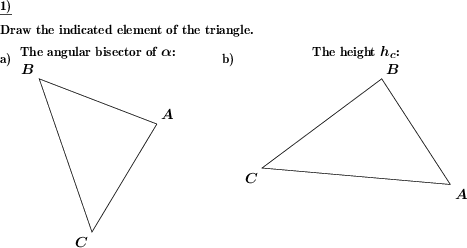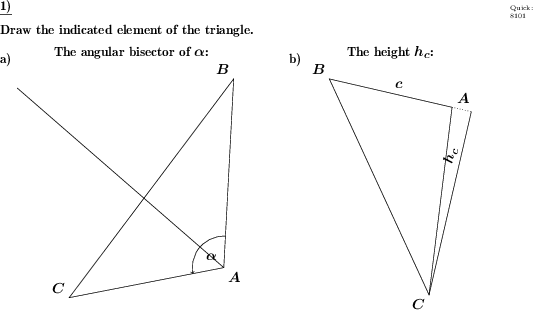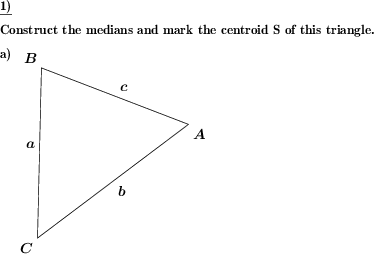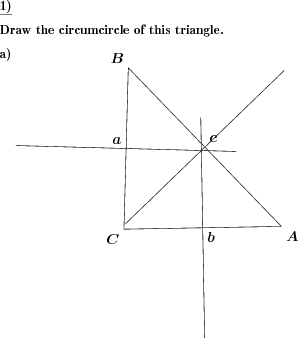Custom math worksheets at your fingertips# Details for problem "Triangle: Draw heights, medians and bisectors"

Quickname: 8101

Junior High School, Middle School, High School.

## Summary

Draw heights, medians, angle bisectors or perpendicular bisectors for a triangle.

## Examples## Description

This a fluency worksheet that will produce heights, medians, and bisectors drawing problems. draw any of the three heights, medians, or bisectors. A triangle is given.

Depending on the problem statement, a specific

• perpendicular bisector
• height
• median
• angle bisector

is to be drawn.

Use this problem in your worksheet to facilitate different abilities in your grades 7 and 8. Use it as a group activity to assign every member a particular problem or to master a particular drawing concept by an individual learner. Practice this worksheet to achieve the following goals.

1. Define each of the heights, medians, and bisectors in a triangle.
2. Identification of heights, medians, and bisectors in a triangle is recalled.
3. Construction of heights, medians, and bisectors will be learned.
4. Describe the process to construct heights, medians, and bisectors in a triangle.

Personalize this worksheet as required in the class or homework to master concepts. The number of problems is configurable. For every type of geometric line, it can be defined whether it can be asked for.

• The number of problems can be selected from 1 to 2.
• Heights, medians, perpendicular bisectors, and angle bisectors can be asked for each worksheet.

Download free printable worksheets for this math problem here. The worksheet contains the problems only, the solution sheet includes the answers. Just click on the respective link.

•Worksheet 1Solution sheet with answers
•Worksheet 2Solution sheet with answers
•Worksheet 3Solution sheet with answers

If you can not see the solution sheets for download, they may be filtered out by an ad blocker that you may have installed. If this is the case, please allow ads for this page and reload the page. The solution sheets will then reappear.

• Do these sample worksheets do not really fit?
• Do you need more math worksheets, with a different level of difficulty?
• Would you like to combine different problems on a worksheet and adjust them to your needs?
• As a teacher, you can put together your own worksheets using the automatically generated math problems provided.
With a free initial credit, you can start creating your own math worksheets in a few minutes.

You can try it for free! Register here, to create custom worksheets now!

## Customization options for this problem

Parameter
Possible values
Number of problems
1, 2
Yes, No
Yes, No
Yes, No
Yes, No

## Similar problems

Remark
Description
Basic view on angle bisectors
Construct the bisector for a given angle using just a compass and a straightedge.
Perpendicular bisectory in general, without triangle context
For a given line segment, construct the perpendicular bisector using only a compass and a ruler.
Perpendicular bisectors in a triangle, in conjunction with the circumcircle
The circumcircle and perpendicular bisectors have to be drawn for a given triangle.
Angle bisectors only, in conjunction with the incircle
The incircle and angle bisectors have to be drawn for a given triangle.
Medians in a triangle, in conjunction with the centroid
The medians and centroid have to be drawn for a given triangle.

## dw-Math worksheet templates that do contain this problem

Name
Title
Description
Label advanced parts of a triangle
Construct perpendicular bisectors, angle bisectors and medians.

## Other types of problems that appear on worksheets with this problem:

Relevance
Name
Description
Quickname
Example
****
Triangle draw incircle
The incircle and angle bisectors have to be drawn for a given triangle.****
Triangle mark centroid
The medians and centroid have to be drawn for a given triangle.****
Triangle draw circumcircle
The circumcircle and perpendicular bisectors have to be drawn for a given triangle.